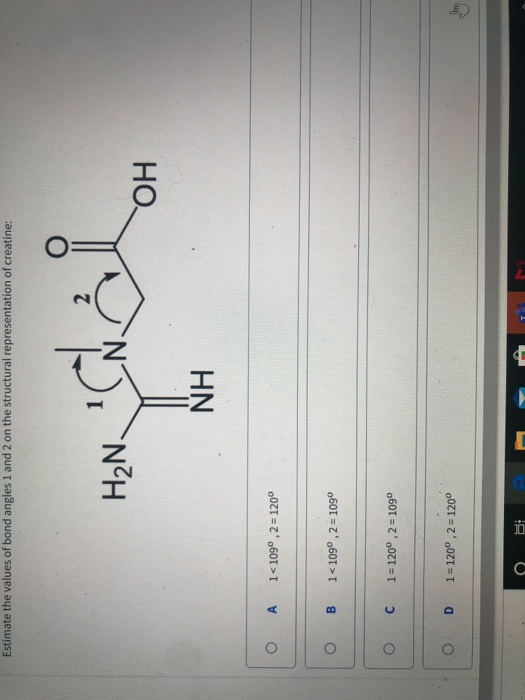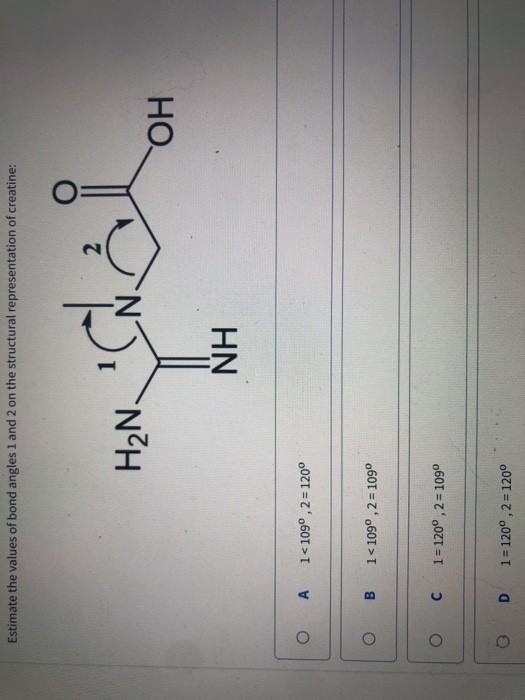# Question Estimate the values of bond angles 1 and 2 on the structural representation of creatine: H₂N I OH NH O A 1<109°,2 = 120° O B 1<109°, 2 = 1090 0 C 1 = 120°, 2 = 109° O D 1= 120°,2 = 1200 Estimate the values of bond angles 1 and 2 on the structural representation of creatine: NH O A 1<109°,2 = 120° O B 1<1090,2 = 1090 C 1 = 120°,2 = 1090 O D 1 = 120°, 2 = 120°